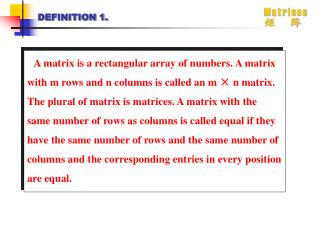Download PresentationDEFINITION 1.DEFINITION 1. - PowerPoint PPT Presentation

Download PresentationDEFINITION 1.
An Image/Link below is provided (as is) to download presentation

Download Policy: Content on the Website is provided to you AS IS for your information and personal use and may not be sold / licensed / shared on other websites without getting consent from its author. While downloading, if for some reason you are not able to download a presentation, the publisher may have deleted the file from their server.

- - - - - - - - - - - - - - - - - - - - - - - - - - - E N D - - - - - - - - - - - - - - - - - - - - - - - - - - -
Presentation Transcript

1. DEFINITION 1. • A matrix is a rectangular array of numbers. A matrix with m rows and n columns is called an m × n matrix. The plural of matrix is matrices. A matrix with the same number of rows as columns is called equal if they have the same number of rows and the same number of columns and the corresponding entries in every position are equal.

2. DEFINITION 2. • Let The ith row of A is the 1 × n matrix [ai1, ai2, …, ain]. The jth column of A is the n × 1 matrix The (i, j)th element or entry of A is the element aij, that is, the number in the ith row and jth column of A. A convenient shorthand notation for expressing the matrix A is to write A = [aij], which indicates that A is the matrix with its (i, j)th element equal to aij.

3. DEFINITION 3. • Let A = [aij] and B = [bij] be m × n matrices. The sum of A and B, denoted by A + B, is the m × n matrix that has aij + bij as its (i, j)th element. In other words, A + B = [aij +bij].

4. DEFINITION 4. • Let A be an m × k matrix and B be a k × n matrix. The product of A and B, denoted by AB, is the m × n matrix with (i, j)th entry equal to the sum of the products of the corresponding elements from the ith row of A and the jth column of B. In AB = [cij], then • Cij = ai1b1j + ai2b2j + … + aikbkj =

5. DEFINITION 5. • The identity matrix of order n is the n × n matrix In = [ ], where =1 if i = j and = 0 if i ≠ j. Hence

6. DEFINITION 6. • Let A = [aij] be an m × n matrix. The transqose of A, denoted by At, is the n × m matrix obtained by interchanging the rows and columns of A. In other words, if At = [bij], then bij = aji for I = 1, 2, … , n and j = 1, 2, … , m.

7. DEFINITION 7. • A square matrix A is called symmetric if A = At. Thus A = [aij] is symmetric if aij = aji for all i and j with 1≤i≤n and 1≤j≤n.

8. DEFINITION 8. • Let A = [aij] and B = [bij] be m × n zero-one matrices. Then the join of A and B is the zero-one matrix with (i, j)th entry aij∨bij. The join of A and B is denoted by A∨B. The meet of A and B is the zero-one matrix with (i, j)th entry aij∧bij. The meet of A and B is denoted by A∧B.

9. DEFINITION 9. • Let A = [aij] be an m × k zero-one matrix and B = [bij] be a k × n zero-one matrix. Then the Boolean product of A and B, denoted by A⊙B, is the m × n matrix with (i, j)th entry [cij] where • cij = (ai1∧b1j)∨(ai2∧b2j)∨…∨(aik∧bkj).

10. DEFINITION 10. • Let A be a square zero-one matrix and let r be a positive integer. The rth Boolean power of A is the Boolean product of r A. The rth Boolean product of A is denoted by A[r]. Hence • A[r] = A⊙A⊙A⊙A⊙A⊙A⊙A⊙A • (This is well defined since the Boolean product of matrices is associative.) We also define A to be In. R times